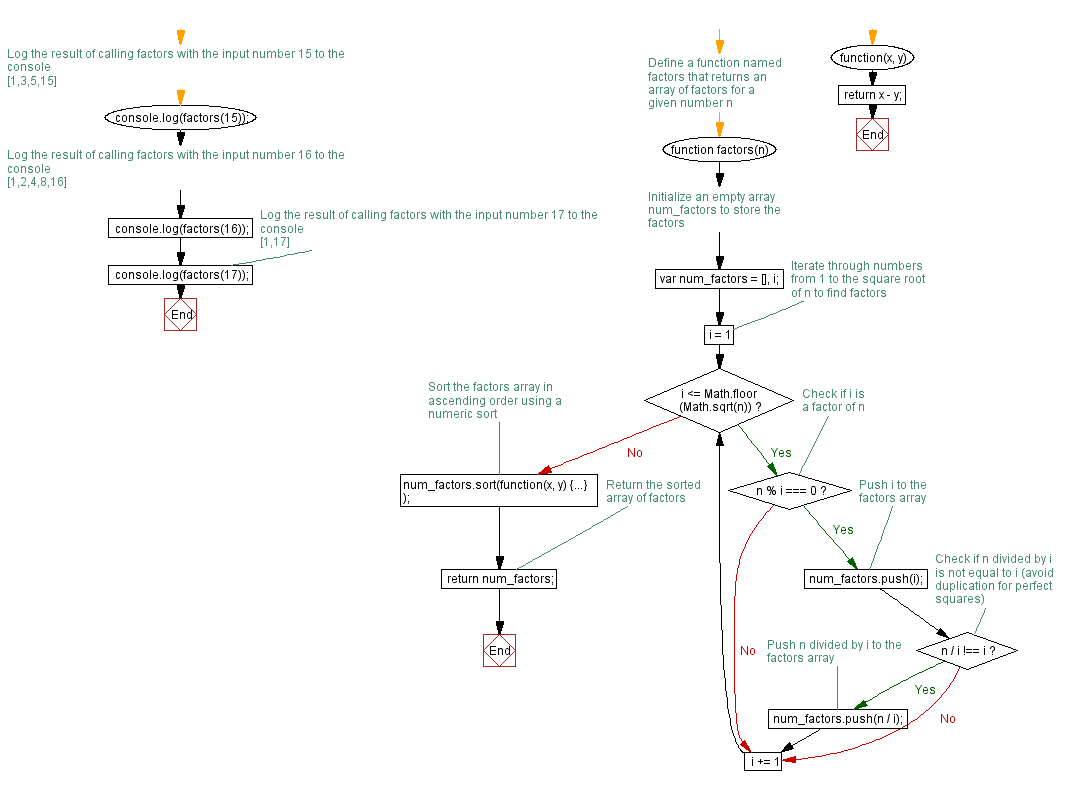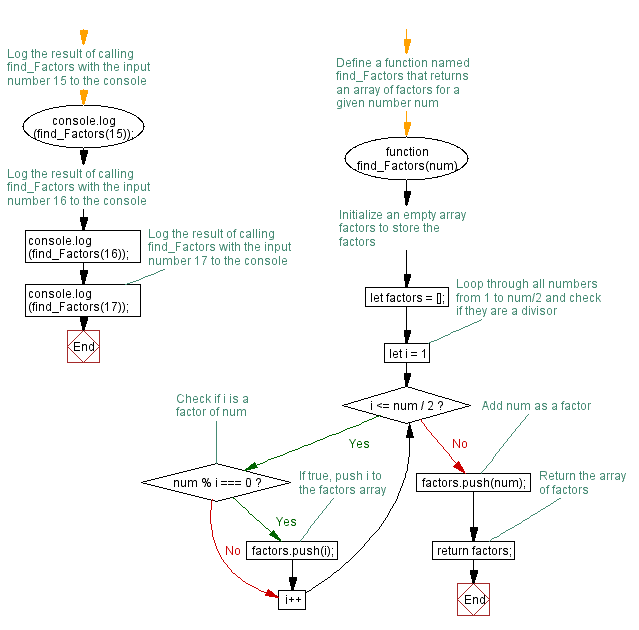# JavaScript: Compute the factors of a positive integer

## JavaScript Function: Exercise-13 with Solution

Write a JavaScript function to compute the factors of a positive integer.

Sample Solution-1:

JavaScript Code:

``````function factors(n)
{
var num_factors = [], i;

for (i = 1; i <= Math.floor(Math.sqrt(n)); i += 1)
if (n % i === 0)
{
num_factors.push(i);
if (n / i !== i)
num_factors.push(n / i);
}
num_factors.sort(function(x, y)
{
return x - y;});  // numeric sort
return num_factors;
}
console.log(factors(15));  // [1,3,5,15]
console.log(factors(16));  // [1,2,4,8,16]
console.log(factors(17));  // [1,17]
```
```

Sample Output:

```[1,3,5,15]
[1,2,4,8,16]
[1,17]
```

Flowchart:Live Demo:

See the Pen JavaScript - Compute the factors of a positive integers-function-ex- 13 by w3resource (@w3resource) on CodePen.

Improve this sample solution and post your code through Disqus

Sample Solution-2:

JavaScript Code:

``````function find_Factors(num) {
let factors = [];
// Loop through all numbers from 1 to num/2 and check if they are a divisor
for (let i = 1; i <= num / 2; i++) {
if (num % i === 0) {
factors.push(i);
}
}

// Add num as a factor
factors.push(num);

return factors;
}
console.log(find_Factors(15));
console.log(find_Factors(16));
console.log(find_Factors(17));
```
```

Sample Output:

```[1,3,5,15]
[1,2,4,8,16]
[1,17]
```

Explanation:
The above function takes a positive integer num as input and loops through all numbers from 1 to num/2 to check if they are divisors of the input number. If a number is a divisor, it is added to an array of factors. Lastly, the function returns an array of factors which includes the input number itself.

Flowchart:Live Demo:

See the Pen javascript-function-exercise-13-1 by w3resource (@w3resource) on CodePen.

Improve this sample solution and post your code through Disqus

What is the difficulty level of this exercise?

Test your Programming skills with w3resource's quiz.

﻿